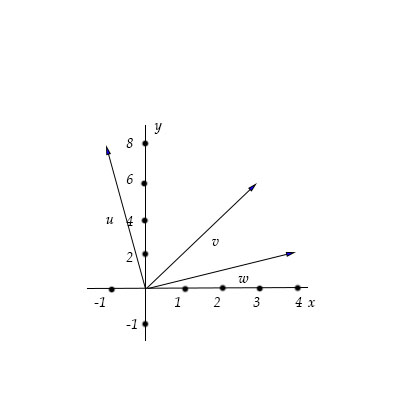# Find the following expressions using the graph below of vector \vec u, \vec v and \vec w. a. \vec...

## Question:

Find the following expressions using the graph below of vector {eq}\vec u, \vec v {/eq} and {eq}\vec w. {/eq}

a. {eq}\vec u + \vec v {/eq}

b. {eq}2 \vec u+\vec w {/eq}

c. {eq}3 \vec v - 6 \vec w {/eq}

d. {eq}|\vec w| {/eq}## Vectors

Geometrically, two vectors can be added by the triangle rule if the two vectors have the same initial point,

then the resultant vector is the vector connecting the terminal points, when the two vector.

To find the resultant angle of two vectors when the components are given, {eq}\displaystyle \mathbf{u}=\langle a_1,b_1\rangle, \mathbf{v}=\langle a_2,b_2\rangle, {/eq}

we will add the vectors by components and obtain {eq}\displaystyle \mathbf{u}+ \mathbf{v}=\langle a_1+a_2,b_1+b_2 \rangle. {/eq}

The magnitude of a vector given by components is {eq}\displaystyle |\mathbf{u} |= |\langle a,b \rangle|=\sqrt{a^2+b^2}, {/eq}

and a multiple of a vector is {eq}\displaystyle n\mathbf{u} = n\langle a,b \rangle=\langle na,nb \rangle. {/eq}

To operate the two-dimensional vectors given in the figure,

we will first obtain the components of {eq}\displaystyle \vec{u}=\langle -1,8\rangle, \vec{v}=\langle 3,6\rangle, \vec{w}=\langle 4,2\rangle. {/eq}

a. To obtain {eq}\displaystyle \vec{u}+\vec{v}, {/eq} we add the components of the two vectors as below

{eq}\displaystyle \vec{u}+\vec{v}=\langle -1,8\rangle+\langle 3,6\rangle=\boxed{\langle 2,14\rangle}. {/eq}

b. To obtain {eq}\displaystyle 2\vec{u}+\vec{w}, {/eq} we add the components of the multiple of {eq}\displaystyle \vec{u} {/eq} and {eq}\displaystyle \vec{w}, {/eq} as follows

{eq}\displaystyle 2\vec{u}+\vec{w}=2\langle -1,8\rangle+\langle 4,2\rangle=\langle -2,16\rangle+\langle 4,2\rangle =\boxed{\langle 2,18\rangle}. {/eq}

c. Similarly, {eq}\displaystyle 3\vec{v}-6\vec{w}, {/eq} is calculated as

{eq}\displaystyle 3\vec{v}-6\vec{w}=3\langle 3,6\rangle-6\langle 4,2\rangle=\langle 9,18\rangle-\langle 24,12\rangle =\boxed{\langle -15,6\rangle}. {/eq}

d. The magnitude of the vector {eq}\displaystyle \vec{w} {/eq} is

{eq}\displaystyle |\vec{w}|=| \langle 4,2\rangle|=\sqrt{4^2+2^2}=\boxed{20}. {/eq}